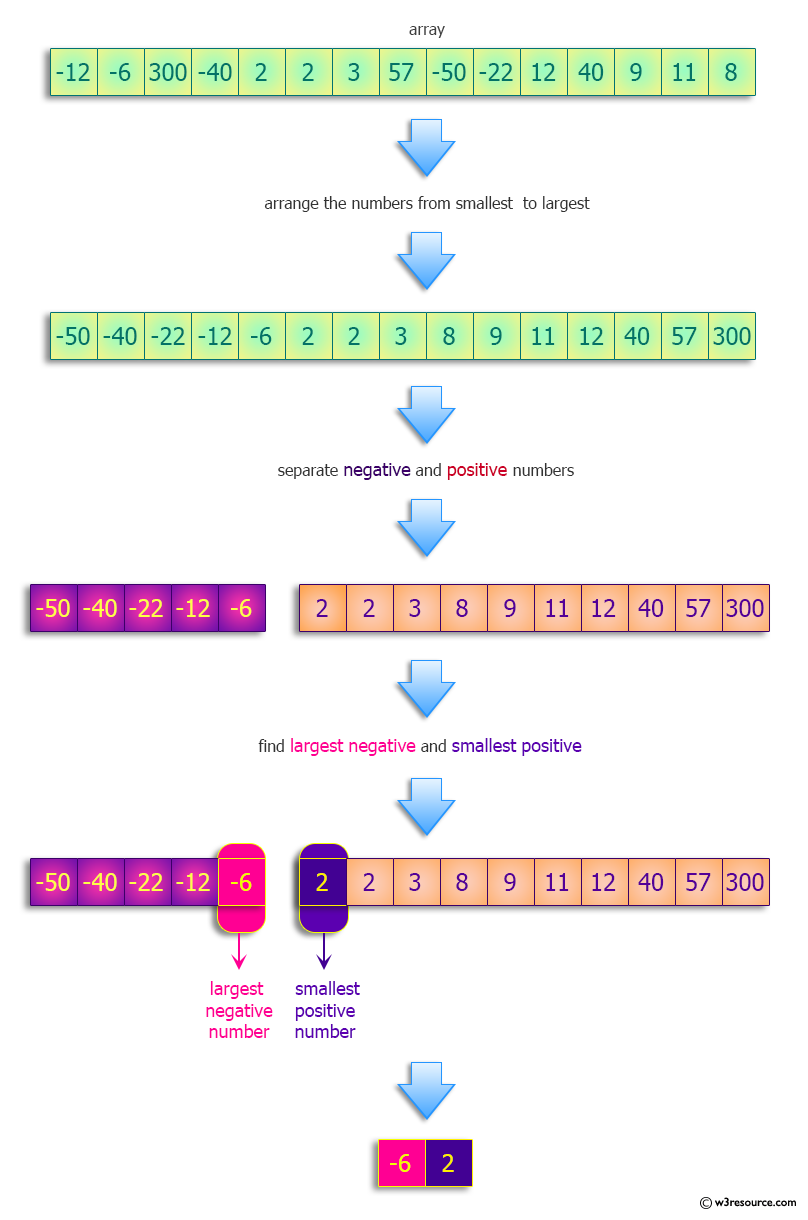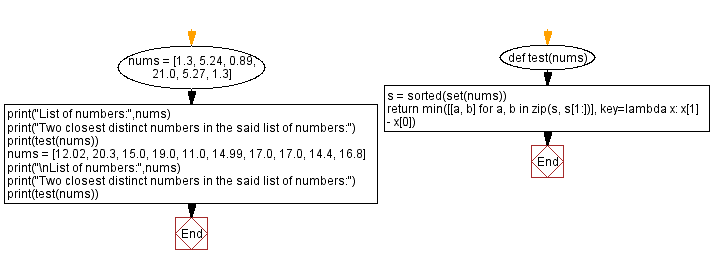﻿ Python: Find the largest negative and smallest positive numbers - w3resource# Python: Find the largest negative and smallest positive numbers

## Python Programming Puzzles: Exercise-79 with Solution

Write a Python program to find the largest negative and smallest positive numbers (or 0 if none).

```Input:
[-12, -6, 300, -40, 2, 2, 3, 57, -50, -22, 12, 40, 9, 11, 18]
Output:
[-6, 2]

Input:
[-1, -2, -3, -4]
Output:
[-1, 0]

Input:
[1, 2, 3, 4]
Output:
[0, 1]

Input:
[]
Output:
[0, 0]
```

Pictorial Presentation:Sample Solution:

Python Code:

``````#License: https://bit.ly/3oLErEI

def test(nums):
pos = [n for n in nums if n > 0]
neg = [n for n in nums if n < 0]
return [max(neg) if neg else 0, min(pos) if pos else 0]
nums=[-12, -6, 300, -40, 2, 2, 3, 57, -50, -22, 12, 40, 9, 11, 18]
print("List of numbers:",nums)
print("Largest negative and smallest positive numbers (or 0 if none) of the said list:")
print(test(nums))
nums=[-1, -2, -3, -4]
print("\nList of numbers:",nums)
print("Largest negative and smallest positive numbers (or 0 if none) of the said list:")
print(test(nums))
nums=[1, 2, 3, 4]
print("\nList of numbers:",nums)
print("Largest negative and smallest positive numbers (or 0 if none) of the said list:")
print(test(nums))
nums=[]
print("\nList of numbers:",nums)
print("Largest negative and smallest positive numbers (or 0 if none) of the said list:")
print(test(nums))
``````

Sample Output:

```List of numbers: [-12, -6, 300, -40, 2, 2, 3, 57, -50, -22, 12, 40, 9, 11, 18]
Largest negative and smallest positive numbers (or 0 if none) of the said list:
[-6, 2]

List of numbers: [-1, -2, -3, -4]
Largest negative and smallest positive numbers (or 0 if none) of the said list:
[-1, 0]

List of numbers: [1, 2, 3, 4]
Largest negative and smallest positive numbers (or 0 if none) of the said list:
[0, 1]

List of numbers: []
Largest negative and smallest positive numbers (or 0 if none) of the said list:
[0, 0]
```

Flowchart:## Visualize Python code execution:

The following tool visualize what the computer is doing step-by-step as it executes the said program:

Python Code Editor :

Have another way to solve this solution? Contribute your code (and comments) through Disqus.

What is the difficulty level of this exercise?

Test your Programming skills with w3resource's quiz.

﻿

## Python: Tips of the Day

Clamps num within the inclusive range specified by the boundary values x and y:

Example:

```def tips_clamp_num(num,x,y):
return max(min(num, max(x, y)), min(x, y))
print(tips_clamp_num(2, 4, 6))
print(tips_clamp_num(1, -1, -6))
```

Output:

```4
-1
```